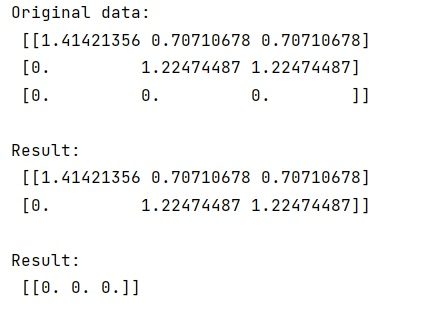# How to remove zero lines from 2-D NumPy array?

Given a 2D NumPy array, we have to remove zero lines from it.
Submitted by Pranit Sharma, on March 14, 2023

## Removing zero lines from 2-D NumPy array

Suppose that we are given a 2D array having pivoted zero-lines at the bottom and we need to split this numpy array in such a way that it divides into two parts; one contains the upper rows that contain non-zero rows and the other contains the zero-lined row.

To remove zero lines from 2-D NumPy array, we can use numpy.all() with an axis argument. We can use this function with indexing the array where we can get the row of zero elements and a row of non-zero with the help of ~ on numpy.all().

Let us understand with the help of an example,

## Python code to remove zero lines from 2-D NumPy array

```# Import numpy
import numpy as np

# Creating a numpy array
arr = np.array([[ 1.41421356,0.70710678,0.70710678],[ 0.,1.22474487,1.22474487],[ 0.,0.,0.]])

# Display original data
print("Original data:\n",arr,"\n")

# Removing zero line
res = arr[~np.all(arr == 0, axis=1)]

# Dispay result
print("Result:\n",res,"\n")

# Getting zero line separately
res = arr[np.all(arr == 0, axis=1)]

# Dispay result
print("Result:\n",res,"\n")
```

### OutputLanguages: » C » C++ » C++ STL » Java » Data Structure » C#.Net » Android » Kotlin » SQL
Web Technologies: » PHP » Python » JavaScript » CSS » Ajax » Node.js » Web programming/HTML
Solved programs: » C » C++ » DS » Java » C#
Aptitude que. & ans.: » C » C++ » Java » DBMS
Interview que. & ans.: » C » Embedded C » Java » SEO » HR
CS Subjects: » CS Basics » O.S. » Networks » DBMS » Embedded Systems » Cloud Computing
» Machine learning » CS Organizations » Linux » DOS
More: » Articles » Puzzles » News/Updates